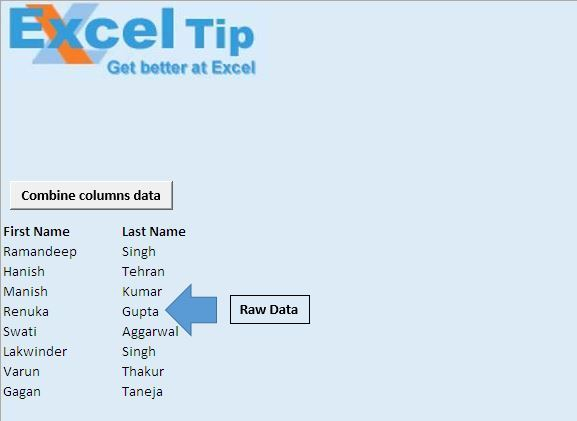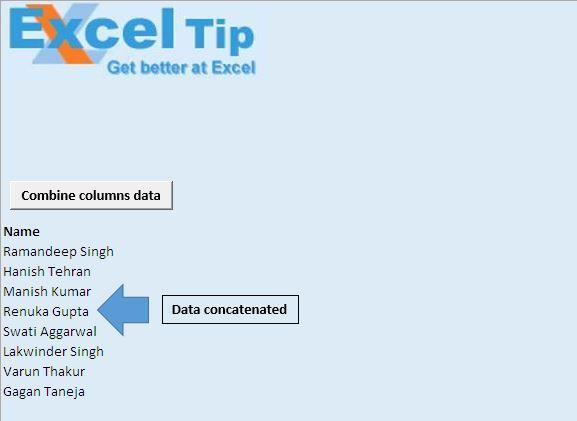# Combine column text and replace using VBA in Microsoft Excel

In this article, we will create a macro to combine text from two consecutive columns to a single column.

Raw data consists of First name and Last name, which we want to merge into a single column.Code explanation

IntRow = Cells(Rows.Count, 1).End(xlUp).Row

The above code is used to get row number of the last cell.

Cells(i, 1) = Cells(i, 1) & " " & Cells(i, 2)

The above code concatenates values from cell in column 1 and column 2.

Columns(2).Delete

The above code is used to delete the second column.```
Option Explicit

Sub CombiningData()

'Declaring variables
Dim i As Integer, IntRow As Long

Application.ScreenUpdating = False

'Getting row number of last cell
IntRow = Cells(Rows.Count, 1).End(xlUp).Row

'Looping from 13th row to last row
For i = 13 To IntRow
'Concatenating the value of two consecutive cells
Cells(i, 1) = Cells(i, 1) & " " & Cells(i, 2)
Next

'Assigning value to cell A12
Range("A12") = "Name"

'Deleting second column
Columns(2).Delete

'Auto adjusting the size of cells in columns
Columns.AutoFit

Application.ScreenUpdating = True

End Sub

```